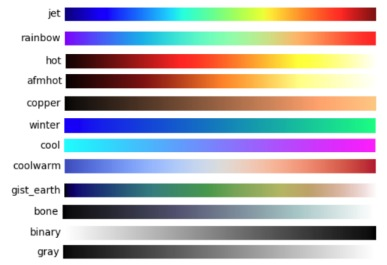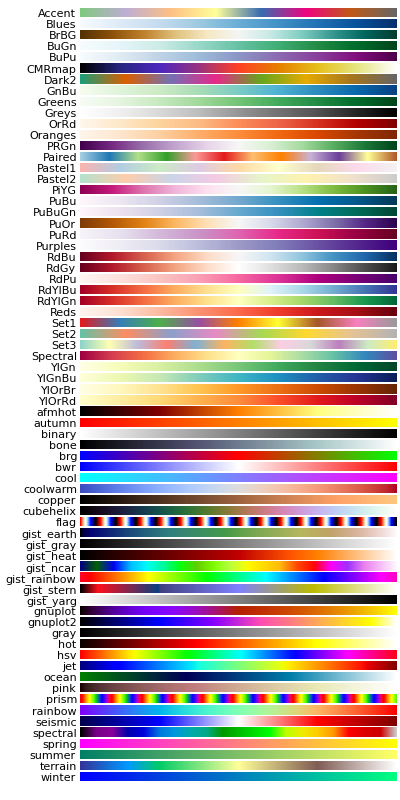# colors¶

Colors definitions and printing methods.

## colorMap¶

vedo.colors.colorMap(value, name='jet', vmin=None, vmax=None)[source]

Map a real value in range [vmin, vmax] to a (r,g,b) color scale.

Parameters
• value (float, list) – scalar value to transform into a color

• name (str, matplotlib.colors.LinearSegmentedColormap) – color map name

Returns

(r,g,b) color, or a list of (r,g,b) colors.

Note

Most frequently used color maps:Matplotlib full list:Tip

Can also use directly a matplotlib color map:

Example
from vedo import colorMap
import matplotlib.cm as cm
print( colorMap(0.2, cm.flag, 0, 1) )

(1.0, 0.809016994374948, 0.6173258487801733)


## getColor¶

vedo.colors.getColor(rgb=None, hsv=None)[source]

Convert a color or list of colors to (r,g,b) format from many different input formats.

Parameters

hsv (bool) – if set to True, rgb is assumed as (hue, saturation, value).

Example

• RGB = (255, 255, 255), corresponds to white

• rgb = (1,1,1) is white

• hex = #FFFF00 is yellow

• string = ‘white’

• string = ‘w’ is white nickname

• string = ‘dr’ is darkred

• int = 7 picks color nr. 7 in a predefined color list

• int = -7 picks color nr. 7 in a different predefined list

## getColorName¶

vedo.colors.getColorName(c)[source]

Find the name of a color.

## kelvin2rgb¶

vedo.colors.kelvin2rgb(temperature)[source]

Converts from Kelvin temperature to an RGB color.

Algorithm credits: tannerhelland

## makeLUT¶

vedo.colors.makeLUT(colorlist, interpolate=False, vmin=None, vmax=None, belowColor=None, aboveColor=None, nanColor=None)[source]

Generate colors in a vtk lookup table.

Parameters
• colorlist (list) – a list in the form [(scalar1, [r,g,b]), (scalar2, 'blue'), ...].

• interpolate (bool) – interpolate or not intermediate scalars

• vmin (float) – specify minimum value of scalar range

• vmax (float) – specify maximum value of scalar range

• belowColor – color for scalars below the minimum in range

• aboveColor – color for scalars above the maximum in range

Returns

the lookup table object vtkLookupTable. This can be fed into colorMap.

Hint

Example: mesh_lut.py

## makePalette¶

vedo.colors.makePalette(color1, color2, N, hsv=True)[source]

Generate N colors starting from color1 to color2 by linear interpolation HSV in or RGB spaces.

Parameters
• N (int) – number of output colors.

• color1 – first rgb color.

• color2 – second rgb color.

• hsv (bool) – if False, interpolation is calculated in RGB space.

Hint

Example: colorpalette.py

## printc¶

vedo.colors.printc(*strings, **keys)[source]

Print to terminal in color (any color!).

Parameters
• c – foreground color name or (r,g,b)

• bc – background color name or (r,g,b)

• bold (bool) – boldface [True]

• italic (bool) – italic [False]

• underline (bool) – underline text [False]

• strike (bool) – strike through text [False]

• dim (bool) – make text look dimmer [False]

• invert (bool) – invert background and forward colors [False]

• box – print a box with specified text character [‘’]

• flush (bool) – flush buffer after printing [True]

• end (str) – the end character to be printed [newline]

Example
from vedo.colors import printc
printc('anything', c='tomato', bold=False, end='' )
printc('anything', 455.5, vtkObject, c='lightblue')
printc(299792.48, c=4)